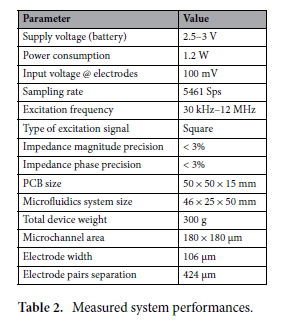## Portable impedance‑sensing device for microorganism characterization in the field

Karim Bouzid 1*, Jesse Greener 2, Sandro Carrara 3 & Benoit Gosselin

## The characterization of microorganisms can be performed using their impedance spectrum, which is a function of the resistivity, dielectric constant, and geometry of the substance under test (SUT). The resistivity and dielectric constant depends on the mobility and quantity of charge carriers in the material41. The impedance is defined from the complex Ohm’s law based on the ratio of a voltage signal to a current signal37, as shown in Eq. 1, where Z is the impedance, V is the applied (or measured) voltage, I is the measured (or applied) current, φ is the phase difference between the voltage and current, ω is the angular excitation frequency, and j is the imaginary unit value. EIS measurement consists in injecting an AC sinusoidal waveform of a known voltage or current to the SUT and measuring its respective output current or voltage response, for a certain number of frequency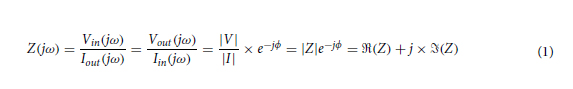## IFC41,44,46,47 is the technique offering the best results in the literature for whole cell characterization so far. It is a label-free non-invasive impedimetric measurement technique based on the Coulter counter48 to measure the volume displacement of particles flowing in a fluid. The particles are detected using IFC by monitoring the impedance changes observed each time a particle passes over electrodes in a narrow channel. This is the case since the particles and fluid have different impedances. Figure 1 describes the principles of IFC. Electrodes are positioned on the walls of a microchannel and the impedance of a liquid flowing within is measured for several excitation frequency. A pulsed waveform resembling the one shown at the bottom of Fig. 1 is thus retrieved for each frequency when a cell flows in the channel. The shape of this pulse depends on the position and relative impedance of the microparticle and fluid in the channel, while its measured module and phase depends on the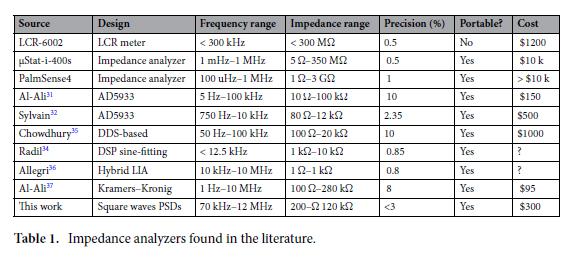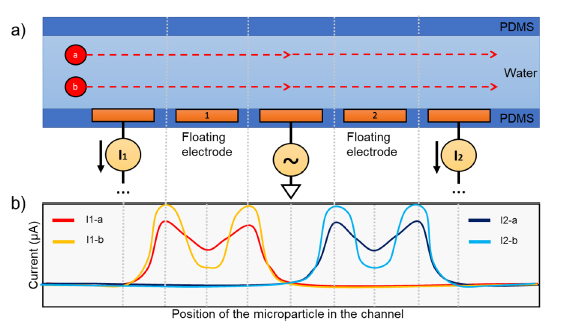## The high volume factor between the cell and detection area when using coplanar electrodes in IFC creates a fringing effect between the electrodes, which is difficult to modelize. A simple empirical equation is given in51 and52 as an alternative to estimate the size of microparticles when using IFC. The particle diameter D can be estimated using a fit to the cubic root of the combined measured impedance magnitude difference |Z1| and |Z2| observed when a particle passes the first and second electrode pairs in the channel, respectively. This is shown in Eq. 2, where G is a constant that accounts for all the parameters linked to the electronics and fluidics, such as the electrode configurations, magnitude and frequency of the excitation signal, filter bandwidth, channel depth and width, electrodes width, EDL capacitance Cedl , buffer conductivity, and electronics gains. The constant G can be determined empirically by testing the IFC system with circular beads of know diameters, then adjusting G until the estimated diameters match the effective beads diameters used during the test.## Impedance‑sensing system. The bloc-diagram of the impedance-sensing device is shown in Fig. 2. It is based on a LIA topology, and extracts the amplitude and phase of a high frequency input signal. It features two channels used to perform a differential analysis. A square waveform with frequency ranging from 200 kHz to 200 MHz is created by the clock-system (Si5351, Silicon Lab). This signal is sent to a quadrature generator to create two 90-degree phase-shifted square waveforms at half the clock signal frequency. The in-phase waveform is attenuated to 100 mVpp to keep a safe linear current response that will not harm the cells during the experiment, and then sent to the two differential electrode pairs of the microfluidics system. Two current responses are obtained, which are then amplified and converted to voltage signals by transimpedance amplifiers (TIAs). The outputs of the TIAs are mixed by two phase-sensitive detectors (PSDs). This yields four output signals representing the real and imaginary current responses of two electrode pairs, which can be sampled by the ADCs. The impedance magnitude and phase differences can be retrieved from the real and imaginary impedance components, and are processed by a square-to-sine spectroscopy algorithm to accurately retrieve the cells properties at a high throughput. The differential design is important to decrease the effects of the common noise and increase the sensitivity to the flowing particles46.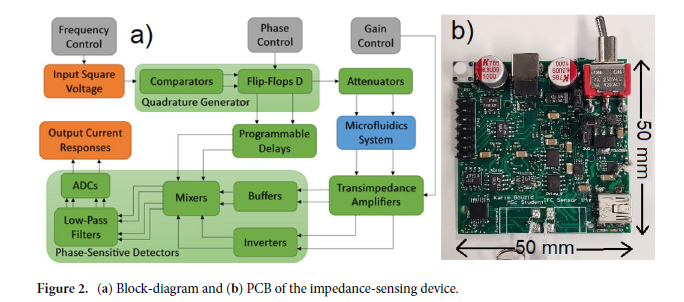## Square input signal. IFC and EIS systems are generally performed using sinewaves. The main advantage of square-waves over sinewaves is that they replace the complex hardware associated with conventional LIAs with much simpler clock-based circuitry54. For instance, the digital-to-analog converter (DAC), wave generator, and linear mixer can all be replaced by a simple clock-system and inexpensive controlled switches. This leads to a decrease of the system’s power-consumption and cost, which are of a prime importance in portable applications. The square wave input voltage Vin outputted by the clock-system and sent to the electrodes is defined in the time domain by Eq. (3). Square signals are multi-frequency signals. Looking at their Fourier transform, we see that a square wave is composed of a fundamental frequency followed by odd-frequency harmonics of decreasing amplitudes. An ideal symmetrical square wave of amplitude 2Vo with peaks at −Vo and +Vo follows the geometrical sum of Eq. (3).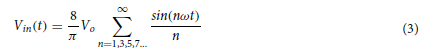## Transimpedance amplifiers. Current responses are obtained from the two electrode pairs that follow Ohm’s law for complex impedance. Current responses being difficult to interact with, two transimpedance amplifiers (TIAs) are used to convert them to voltage signals. TIAs are current to voltage converters generally implemented using one operational amplifier, as shown in Fig. 3. The practical implementation also uses a capacitor for stability in parallel with the resistor in the feedback loop. In the simplest case for a square input signal, Eq. (4) represents the voltage output of the TIAs in time VTIA(t) , where |Z| and θ are respectively the impedance magnitude and phase of the SUT for any harmonic angular frequency nω0.## To accommodate for a wide range of input impedances, a programmable gain array (PGA) with a feedback resistor Rf and capacitor Cf is added to the TIAs to control the gain at will. The PGA is achieved using a multiplexer toggled by a microcontroller that can switch between different gain resistors in the feedback loop of the TIAs30. The feedback capacitor is needed by the TIAs to prevent high-frequency ringing. This can cause a limitation for high-frequency measurements since attenuation is expected at frequencies higher than a couple of megahertz because of the time constant of the RC network formed by Rf and Cf. However, the prototype can still be used at higher frequencies with adequate calibration, although with a reduced accuracy associated with the lessened measured signal amplitude. The trade-offs associated with TIAs are described in Orozco55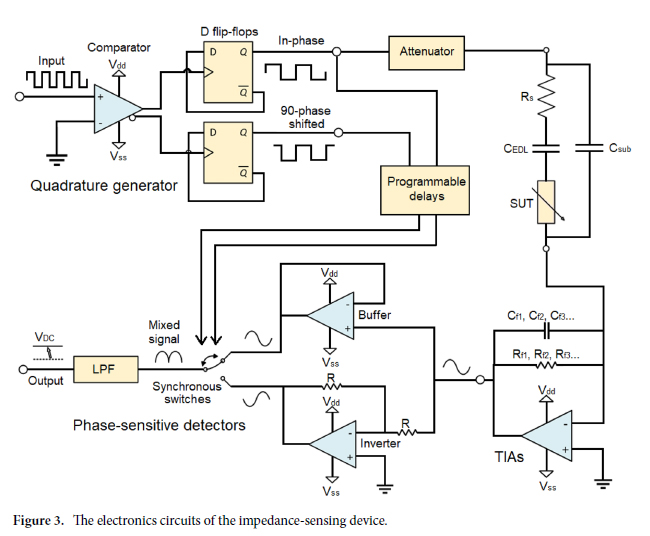## Phase‑sensitive detectors. The TIAs outputs cannot be sampled directly using an ADC since the frequency of the signals of interest is too high (the relevant harmonics can go as high as 110 MHz considering the five first harmonics of a 10 MHz square signal). A solution consists of using a mixing and filtering stage implemented from a phase-sensitive detector (PSDs). PSDs act as narrowband filter similarly to LIAs to precisely retrieve the amplitude and phase of a signal buried in noise41. PSDs use square signals and an inverter to switch between the original and inverted version of the signal of interest at the frequency of the square reference signal. This switching yields DC components proportional to the real and imaginary current of the SUT’s impedance. The behavior and implementation of the PSD is shown in Fig. 3. The DC values of the real and imaginary components of the current responses at the output of the PSDs are described by Eqs. (5) and (6).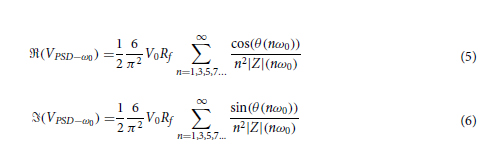## Square to sine spectroscopy algorithm. Now, as can be seen from Eqs. (5) and (6), it is not trivial to recover the impedance magnitude and phase of the fundamental when using PSDs, as is the case with LIAs. Indeed, harmonics of the square excitation signal are present at every odd frequency of the fundamental which adds a systematic error to the impedance measurement54,56. The harmonics present in the square signal are multiplied together by the mixer and pushed to DC along with the desired fundamental frequency. This systematic error is non-trivial as it depends on the impedance response of the SUT. An algorithm inspired by Subhan54 can be used to cancel the systematic error. The values of square impedance at the harmonics can be subtracted or added to the fundamental impedance following a certain set of rules described in Subhan54. It is thus necessary to measure the entire impedance spectrum before computing the corrected impedance at a given frequency. The real component of the impedance devoid of the systematic error Vsine−ω0 , follows Eq. (7)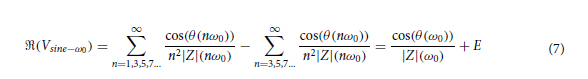## where E is the residual error after correction, which depends on the number of frequency points that were subtracted. There is, however, a practical limit to the number of points that can be subtracted considering that the impedance at that frequency must be measured (or extrapolated) beforehand, which might not be possible for high frequency samples. A similar process can be repeated for the quadrature component in Eq. (8). The corrected impedance magnitude and phase of Eqs. (9) and (10) can then be reconstructed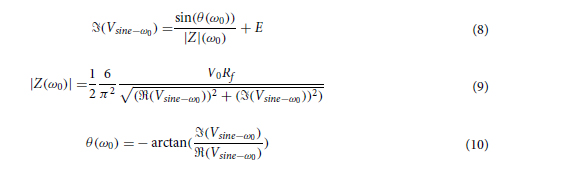## The whole fabrication process is described in Bouzid38. A mold is initially drawn on a CAD software such as Solidworks. The drawn model is sent as a .STL file to the CADworks3D software to be meshed. This new meshed model is used by the stereolithography 3d-printer CADworks3d H50-405 to print a 3D-mold using Master Mold for PDMS Device Photopolymer Resin TM. The resin is then rinsed with IPA (90%) or methyl hydrate for 5 min and blow dried using an air gun. The mold is then cured using UV light around 400 nm in a LED light curing box for 50 min. PDMS ( SYLGARDTM 184 Silicone Elastomer Base) and a curing agent ( SYLGARDTM 184 Silicone Elastomer Curing Agent) are mixed at a ratio of 10:1 and degassed by letting the solution rest for 60 min. The mixture is put in the mold and cooked on a hot plate for 50 min at 70◦ , then dried overnight at 40 ◦C58. A scalpel is used to gently prick-off the channel from the mold. The surfaces of the PDMS microchannel are then exposed to plasma at 600 KPa for one min. Polyethylene glycol is immediately applied on the surface to keep its hydrophilicity for longer periods of times. After a 10 min wait time, the PDMS microchannel is cooked for 10 min at 130 ◦ C on a hot plate58. A dry gun is blown on the channel to take away any residues. The PCB electrodes are aligned on the microchannel and a pressure is applied to seal them. The PDMS channel and PCB electrodes are sealed tight by a system of 3d-printed squeezers that compress the channel and PCB electrodes. Those are tightened together by bolts, hermetically sealing the microchannel and PCB electrode due to the flexible nature of PDMS. Finally, tubes are inserted at the inlet and outlet of the PDMS microchannel, concluding on the whole process. The microfluidics system is thus hermetic and easy to handle.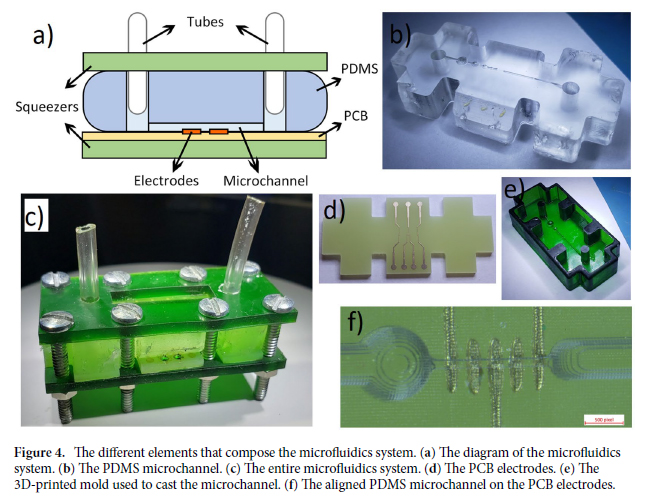## The electrodes in this study are fabricated on a one-layer PCB. They have a size of 46 × 21 × 1.6 mm. The PCB uses the conventional substrate FR-4 TG130 in the exact shape of the microfluidics channel. The employed surface finish is immersion gold (ENIG) (1U”) with 1 oz copper. Inert metals such as gold or platinum are used for the electrodes because of their convenience in casting for small dimensions, for their unlimited lifetime, and since other types of electrodes such as Ag/AgCl are unsuitable for high excitation frequency28. The electrodes are 101.6 μm wide and are separated by 101.6 μm each. The PCB electrodes and their alignment with the microchannel are shown in Fig. 4.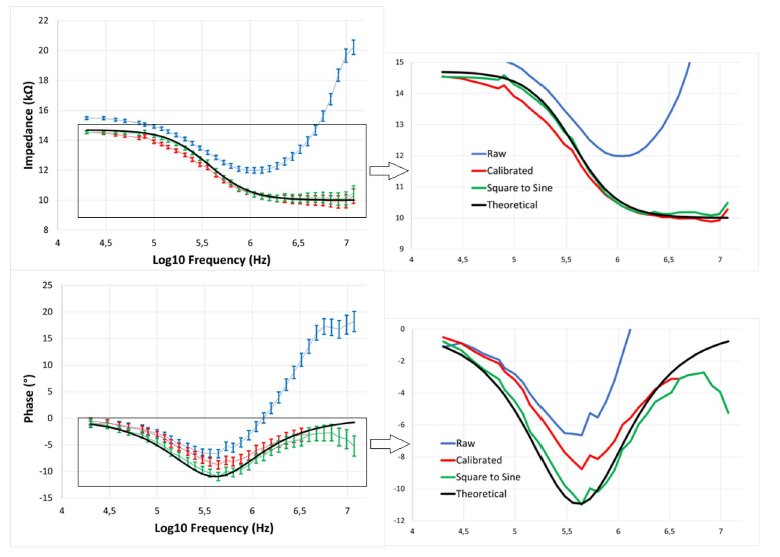## Collected datasets. The true potential of IFC sensors lies in how automatizable the sampling and testing process can be for biological studies. It could be imagined that a team of biologists collects the impedance data of millions of cells and particles using the portable device described in this study, and efficiently extract the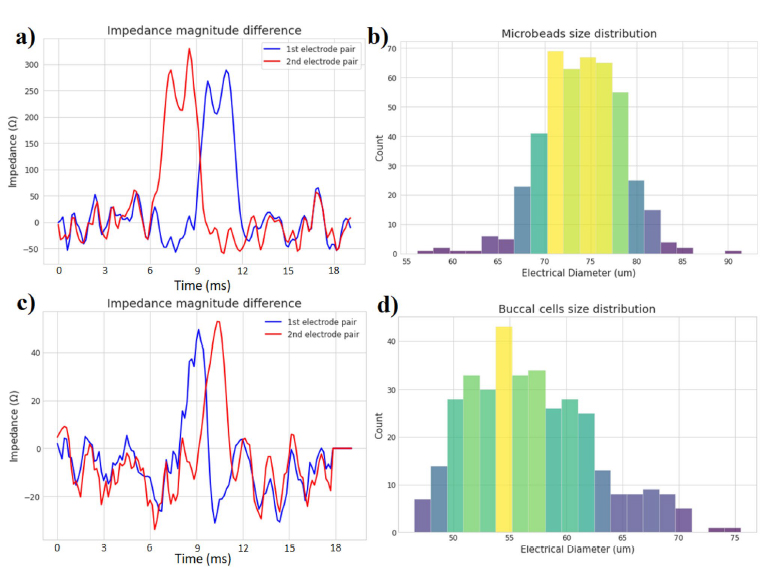## Measured performance. The performance and characteristics of the presented impedance-sensing device and microfluidic system are summarized in Table 2. The impedance-sensing system created for this study is the first found in the scientific literature to achieve great sensitivity level over wide frequency and impedance range while boasting a small size, low-cost, and low power-consumption. The impedance-sensing device coupled with the microfluidics systems are effectively capable of measuring and estimating the properties of the microparticles of sizes going as low as 45 μm when used within a 180 μm wide microchannel. The dimensions of the microchannel are fixed by the limitations of the 3D-printer, which could be improved for this study by using a 3D-printer such as the one designed by Gong63. This homemade 3D-printer is specifically made for microfluidics and can attain truly microscopic scales of 18 × 20 μm by modifying the type of resin used and optimizing the stereolithographic process. This higher resolution would help increase the sensitivity of our device for smaller particle detection. The impedancesensing device takes 50 mm × 50 mm × 15 mm of space, while the microfluidics system is 46 mm × 25 mm × 50 mm, with a combined weight of 300 g, making them portable enough to be put in a backpack for applications in the field. The electrode pairs in the microchannel are separated by 424 μm and each have a width of 106 μm compared to the microchannel size of 180 μm × 180 μm. The impedance-sensing system only needs 1.2 W to